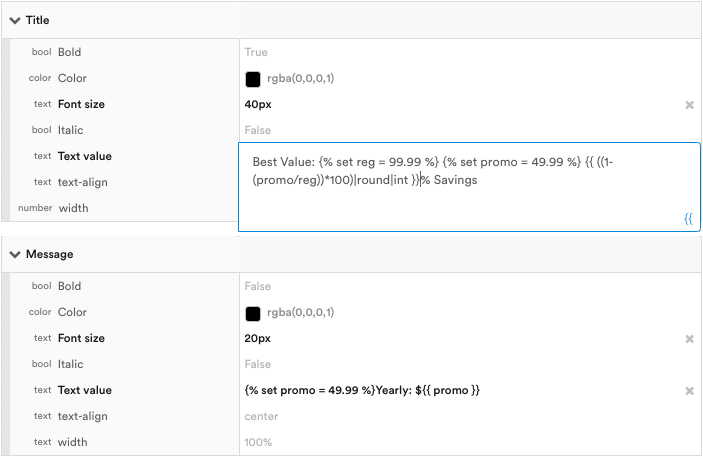# Doing math with dynamic values

Use Jinja-based templating to do mathematical equations automatically in messages

You can use Jinja-based templating to conduct mathematical equations within Leanplum messages.

To set this up in a message:

1. Place your cursor at the point within the ‘Message’ or ‘Title’ text field where you want to add the Jinja formatting.
2. Insert your Jinja code directly into the text field.To conduct math in Jinja, first we have to set the variables. For example:

``````{% set X = 5 %}
{% set Y = 10 %}
``````

Once we've set our variables, we can create the following equations:

``````{# Division #}
{% set X = 5 %} {% set Y = 10 %} {{ X/Y }}

{# Multiplication #}
{% set points = 5 %} {% set multiplier = 10 %} {{ X*Y }}

{% set var1 = 5 %} {% set var2 = 10 %} {{ X+Y }}

{# Subtraction #}
{% set X = 5 %} {% set Y = 10 %} {{ X-Y }}
``````

📘

Preventing 3.14159265359...

To prevent numbers with many decimal places, pipe your results through `round` and `int`. See the example below for details.

## Example: Calculating % off regular price

For example, let’s say we want to customize an in-app message by inserting the value of a product’s original price, then showing the percentage the user could save if they opt-in to your current promotional offer:

• Yearly Regular Price: \$99.99
• Yearly Promotional Price: \$59.99

To this up in a message, first we'll set our variables:

`{% set reg = 99.99 %} {% set promo = 49.99 %}`

Next, we can create the equation like so:

`{{ ((1-(promo/reg))*100)|round|int }}` % Savings

The result should look something like this:

It is important to pipe the results to `round` and `int` as shown above, or else your result might look something like:
`Round` will round the number to a given precision. The first parameter identifies the precision (default of 0). The second is the rounding method, which if not specified will default to ‘common’ (which rounds either up or down).